Successfully reported this slideshow.
We use your LinkedIn profile and activity data to personalize ads and to show you more relevant ads. You can change your ad preferences anytime.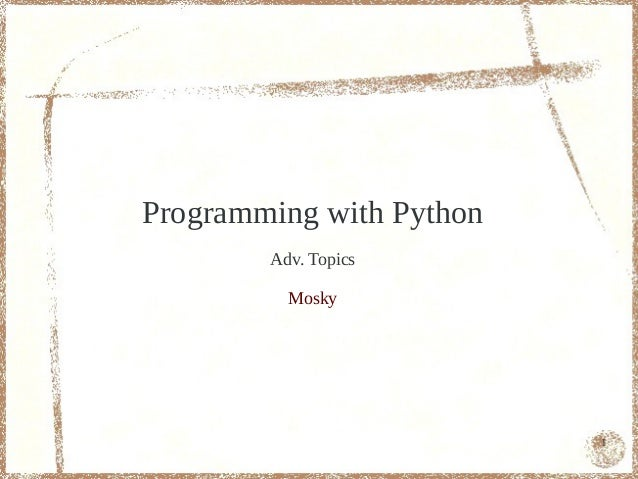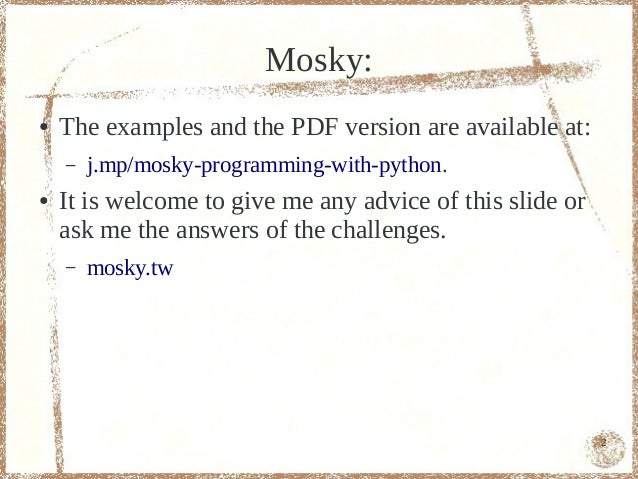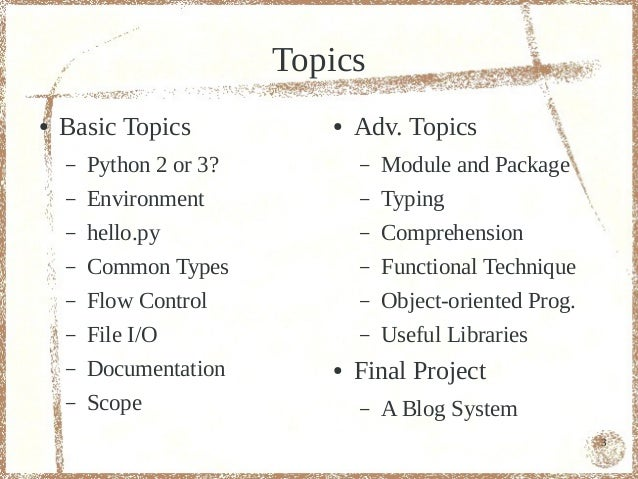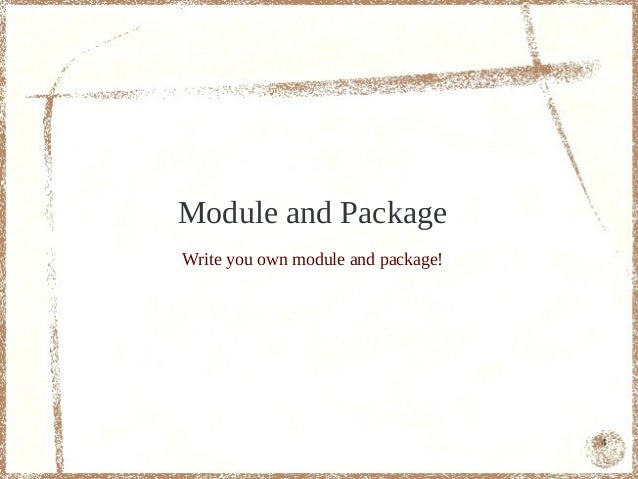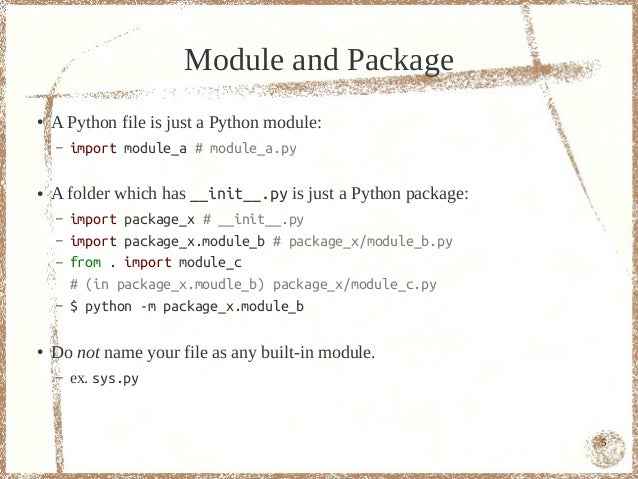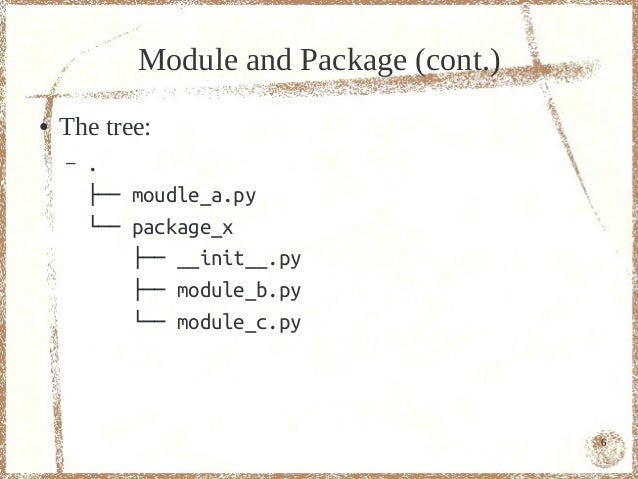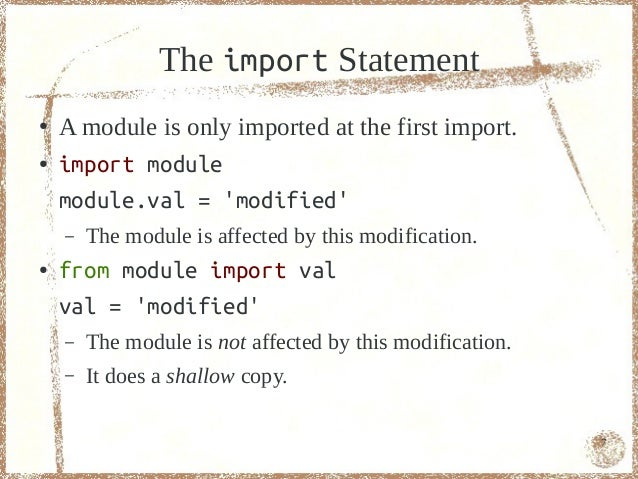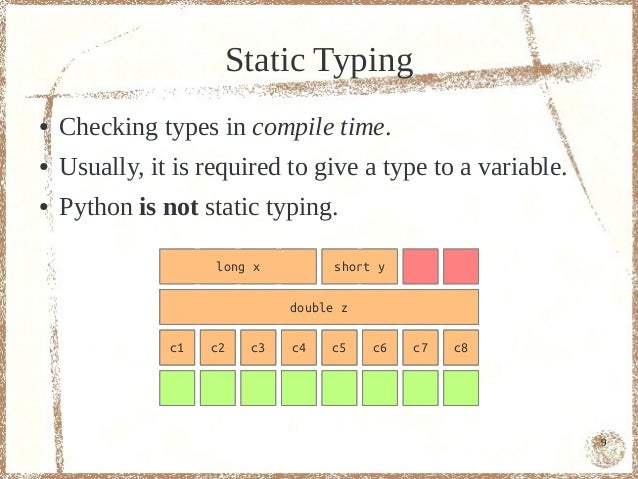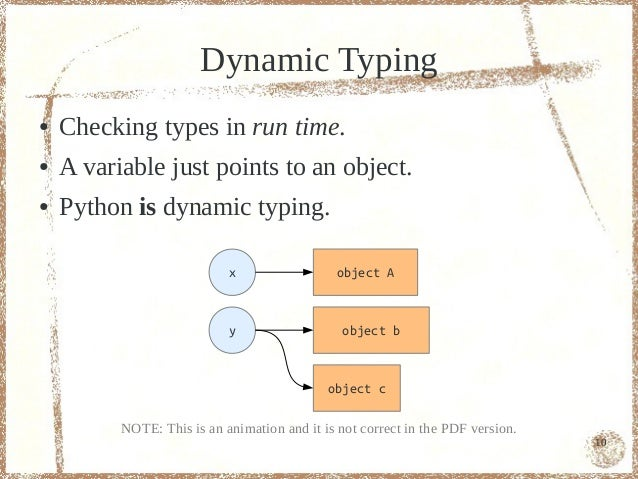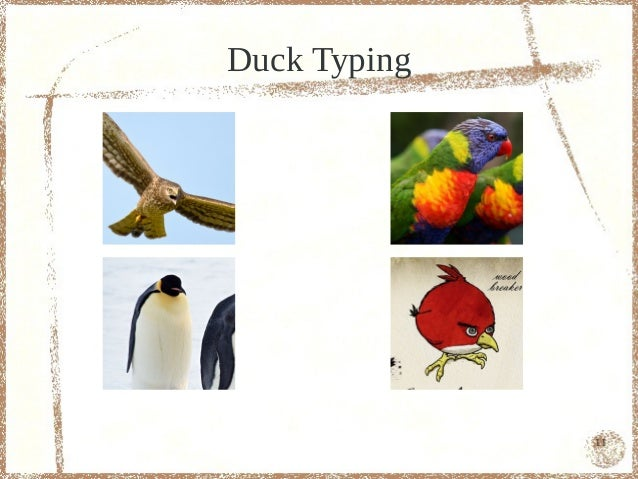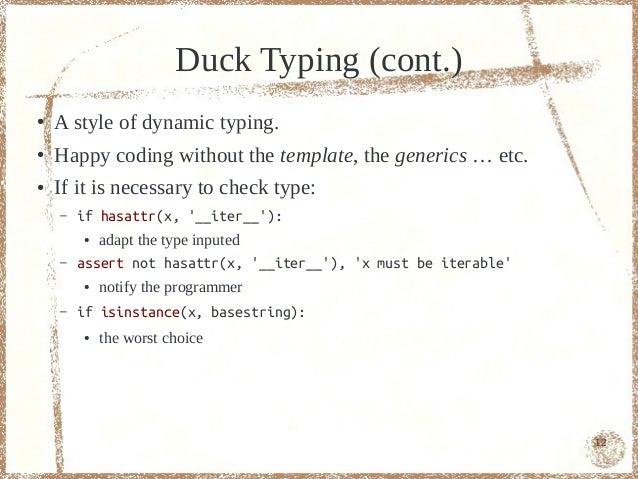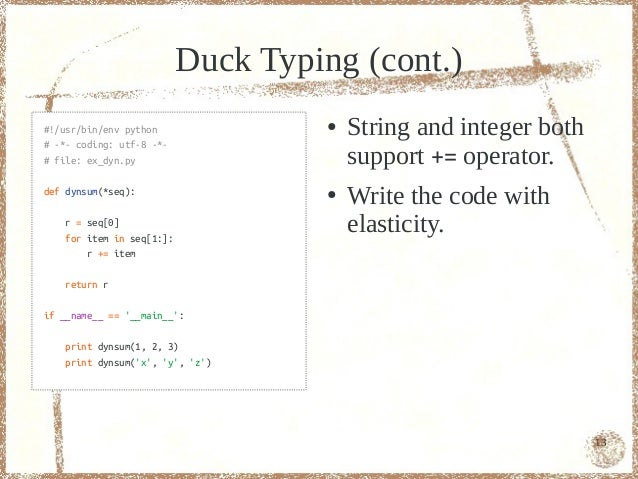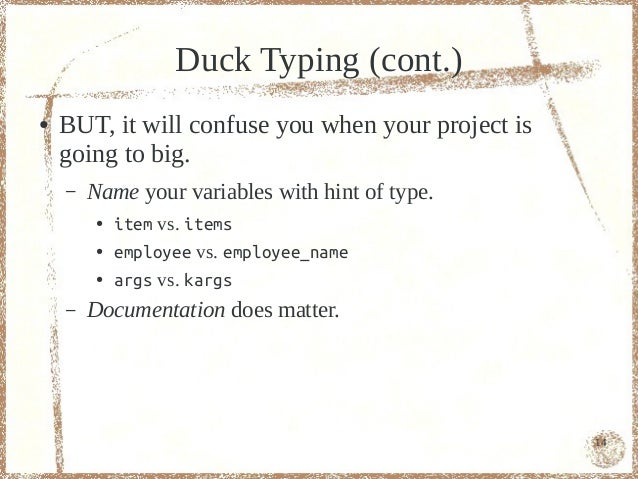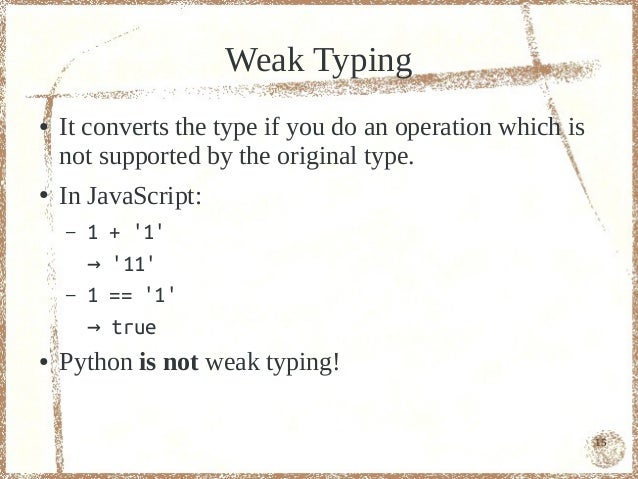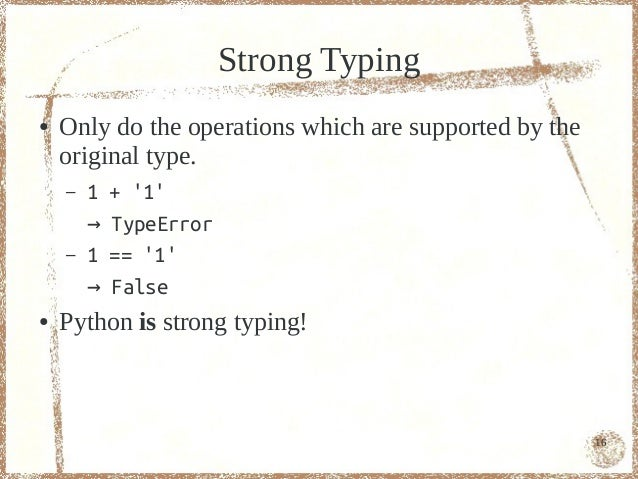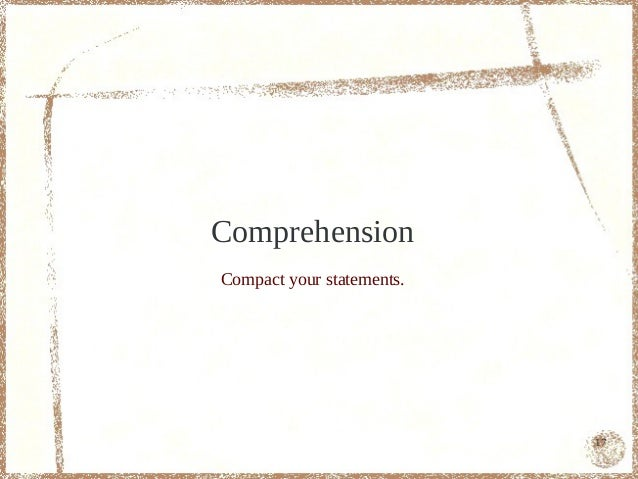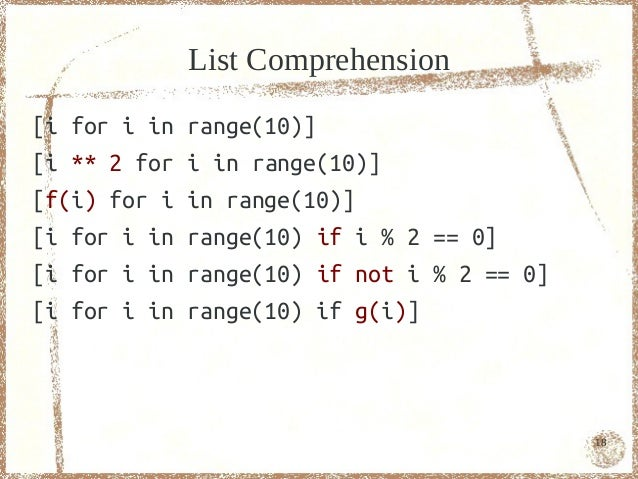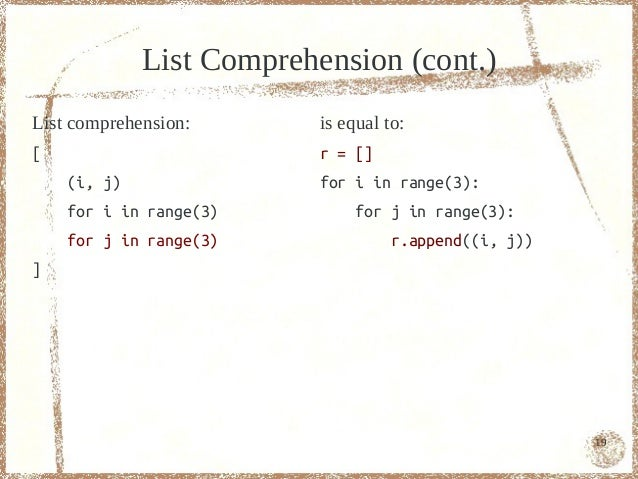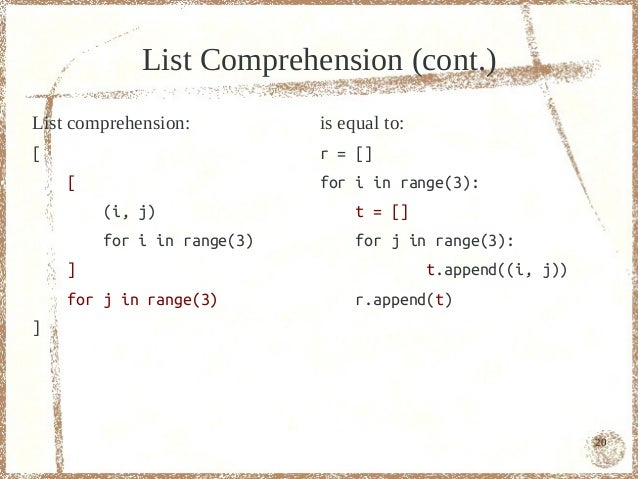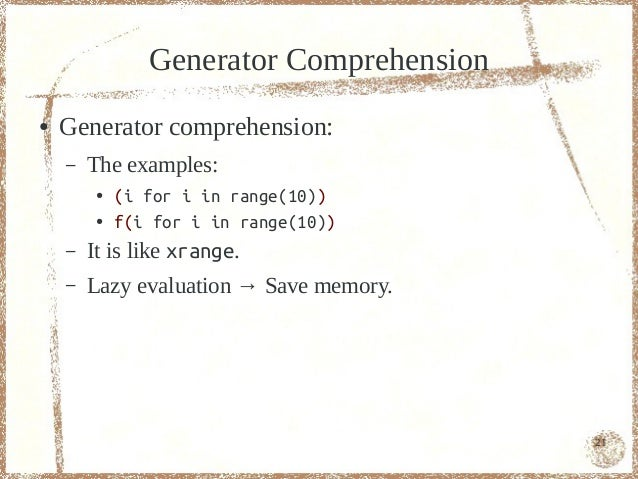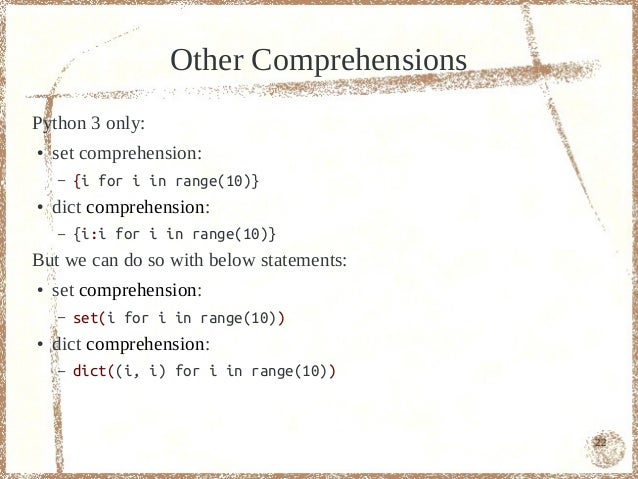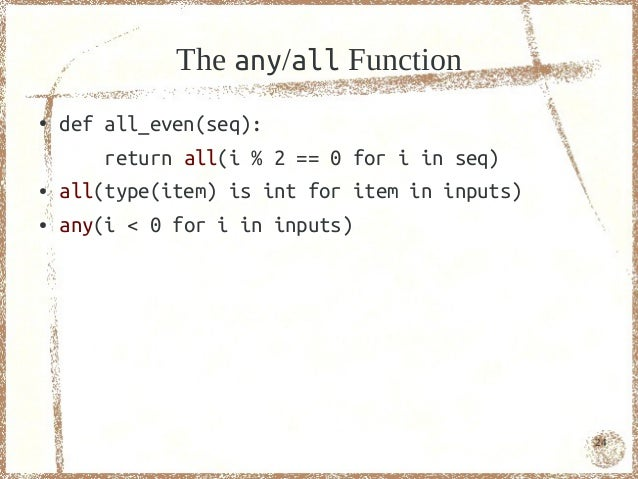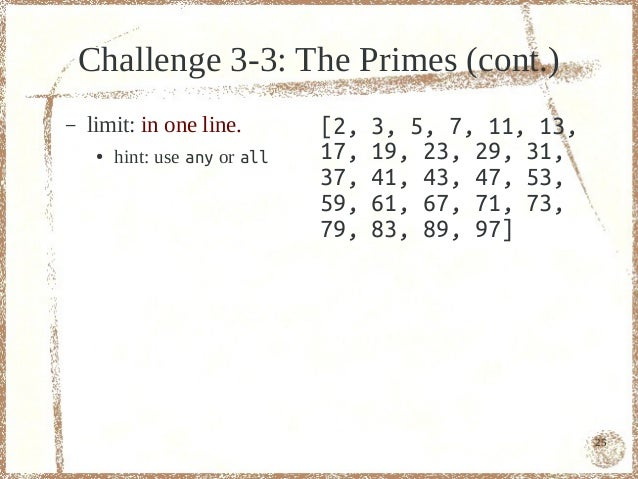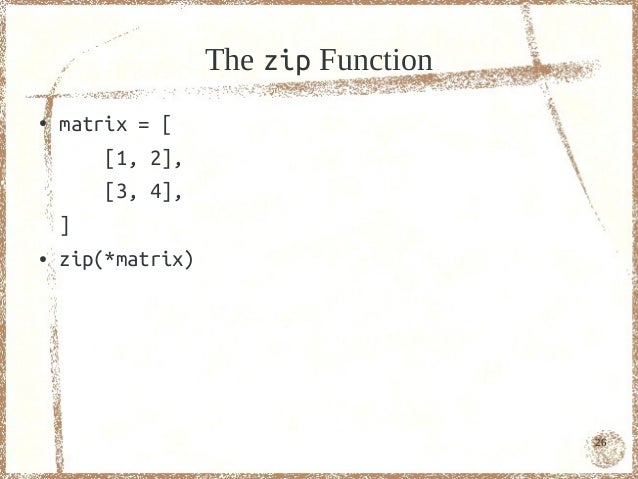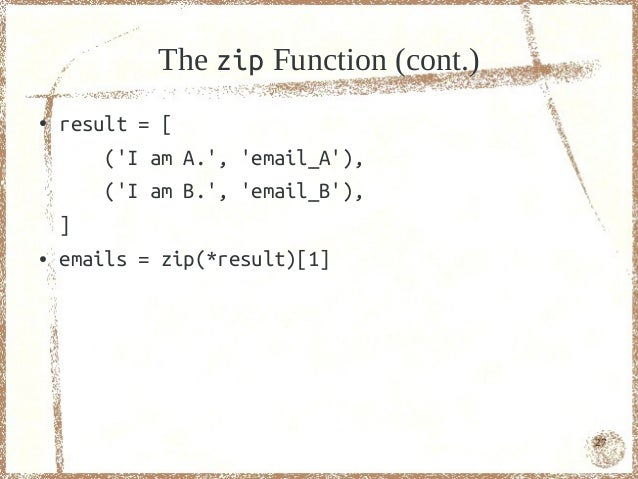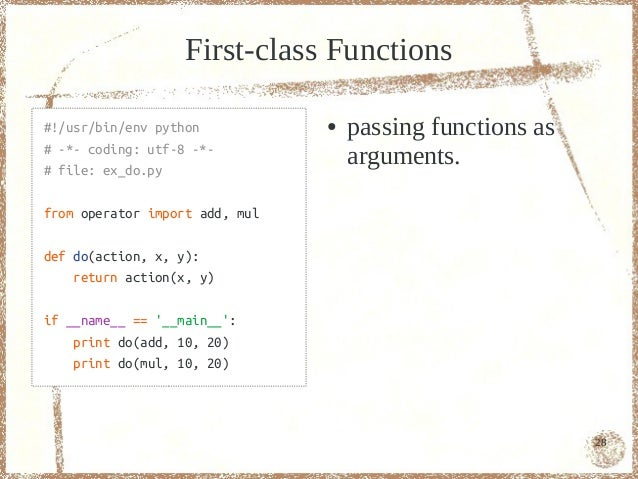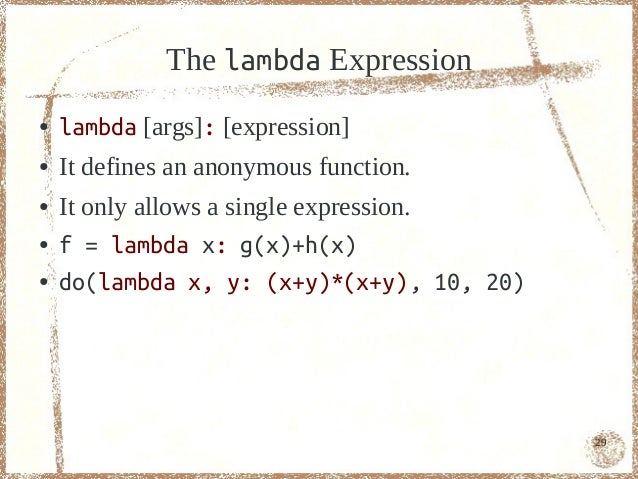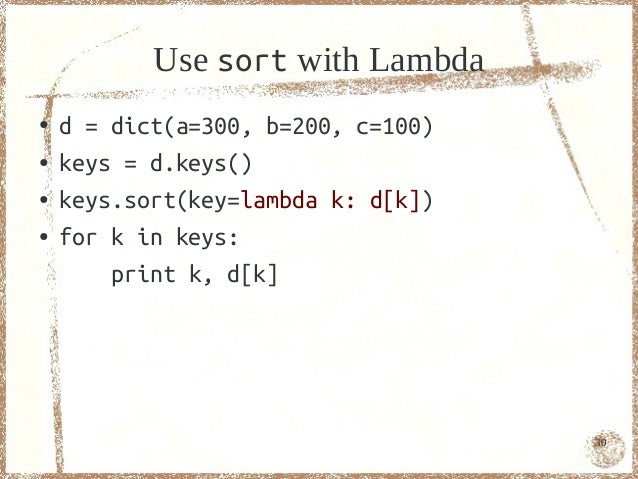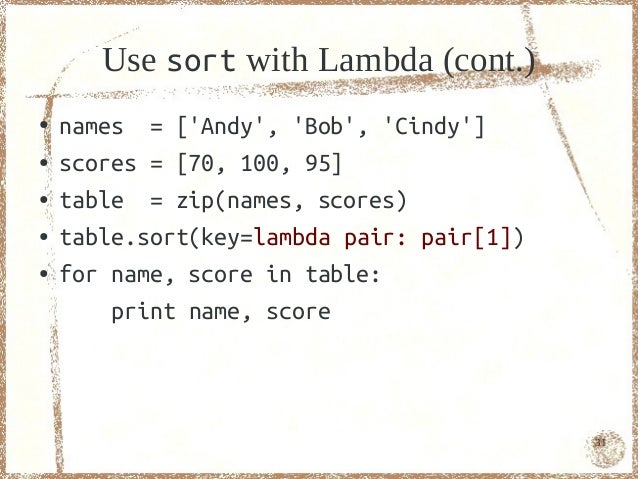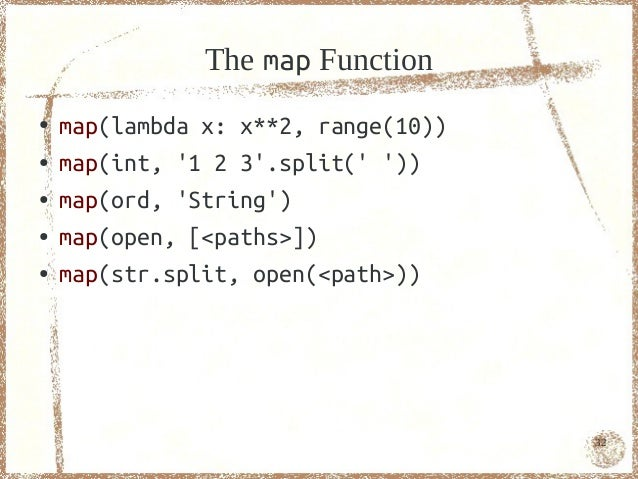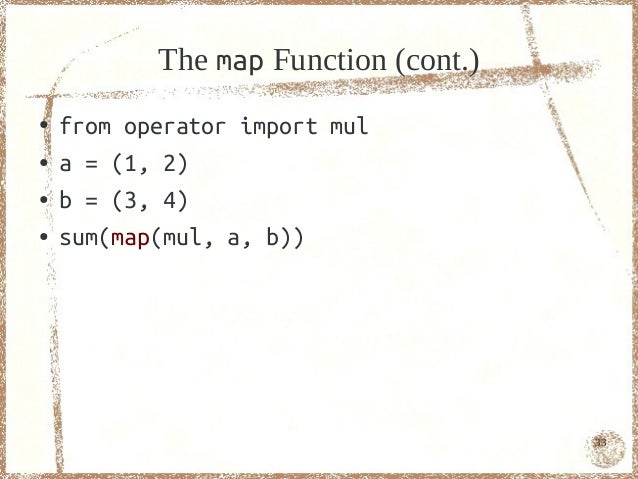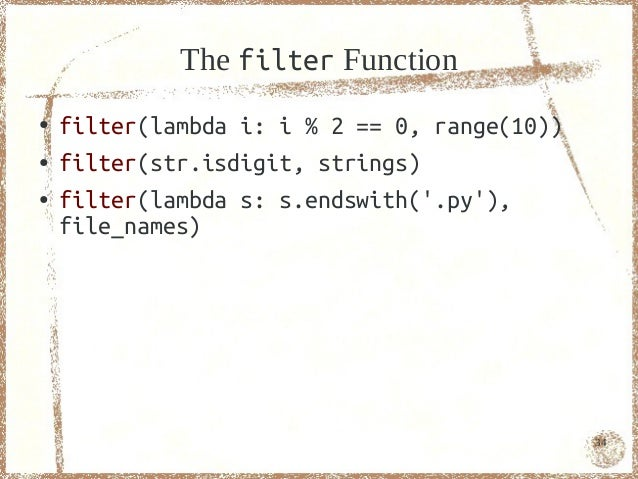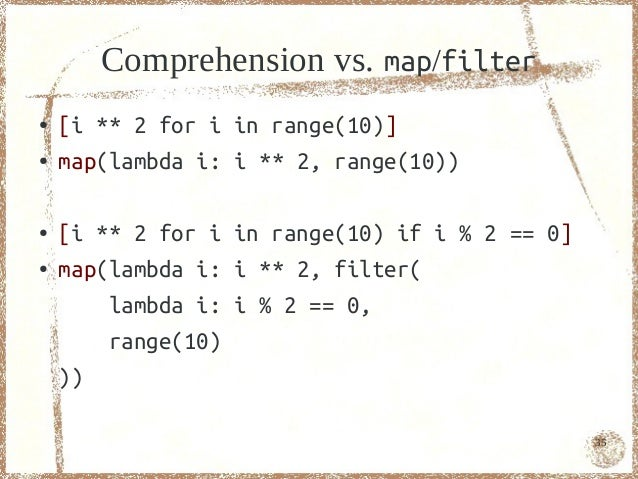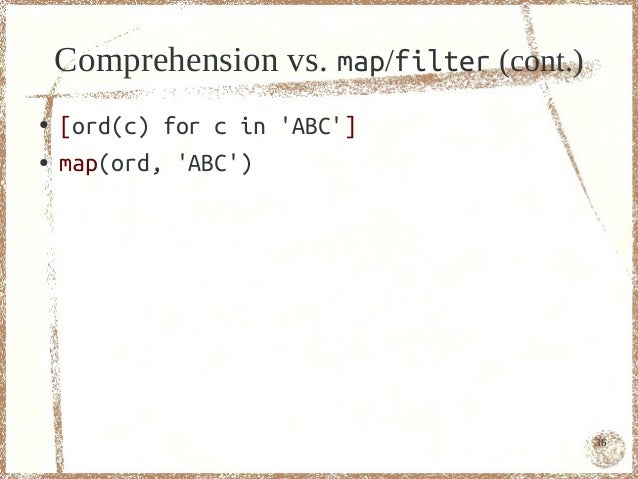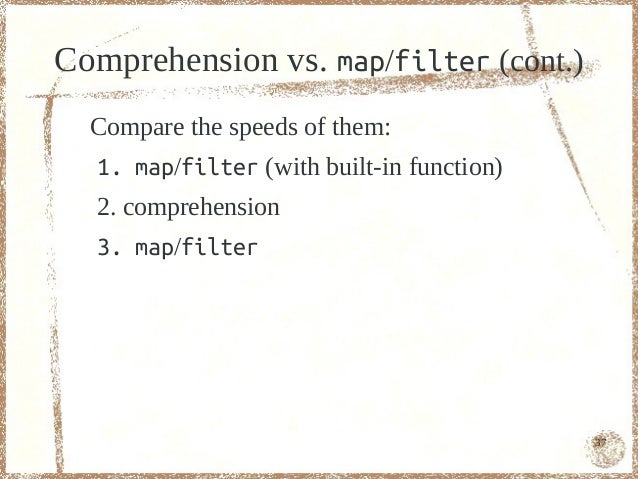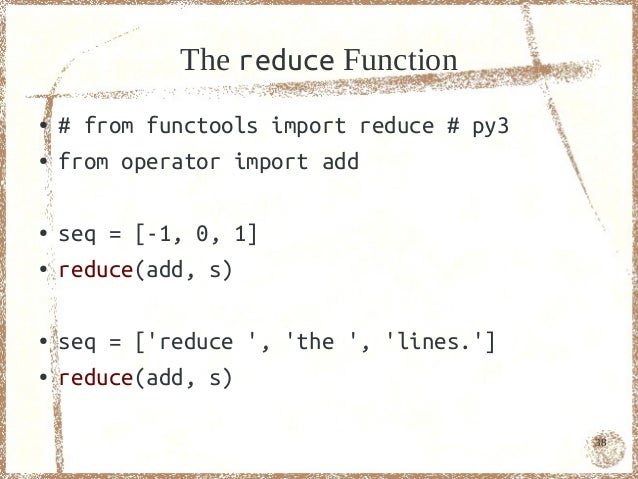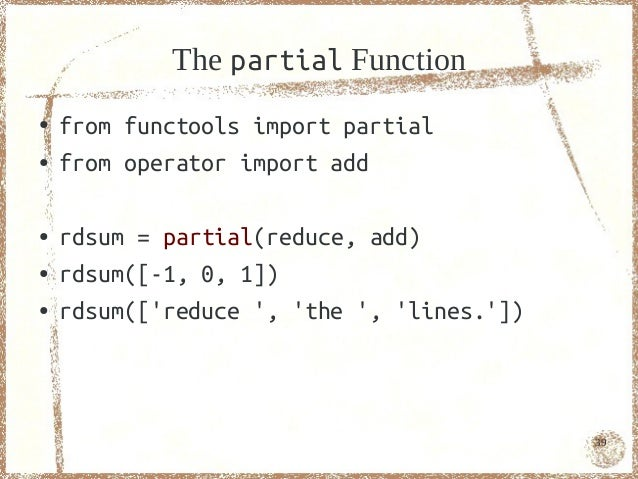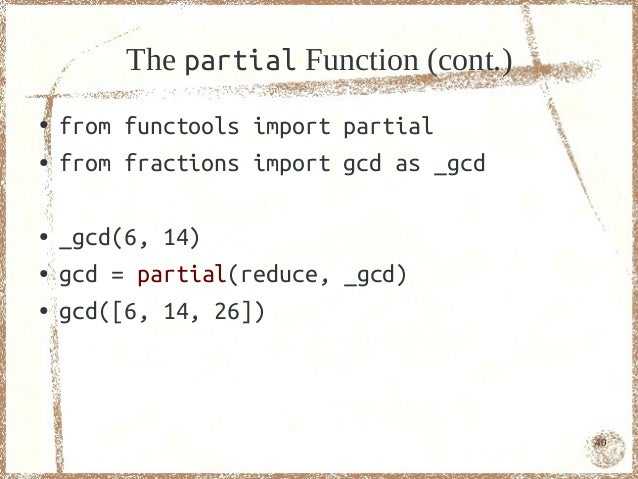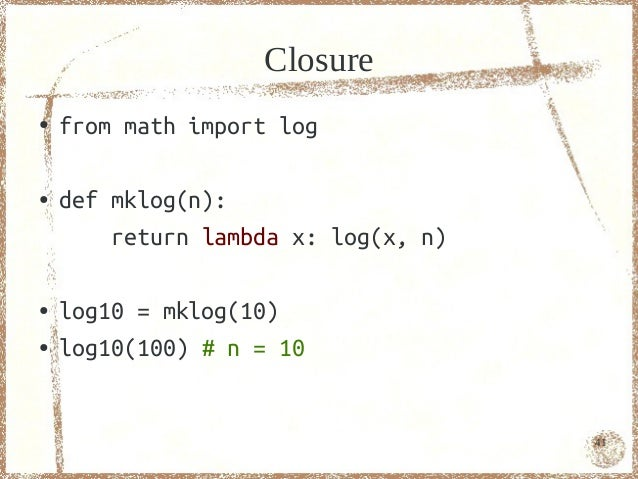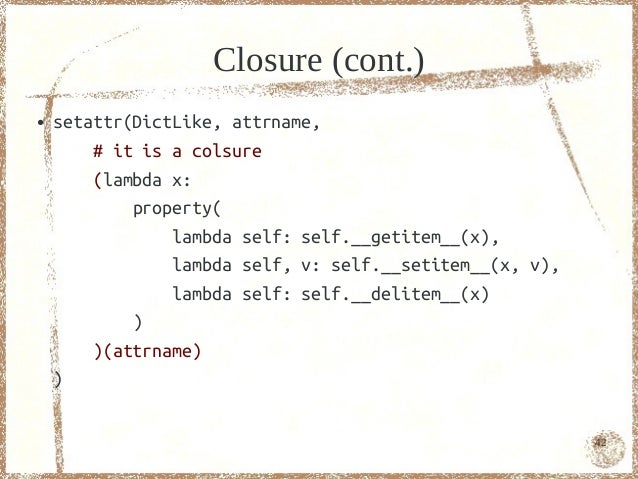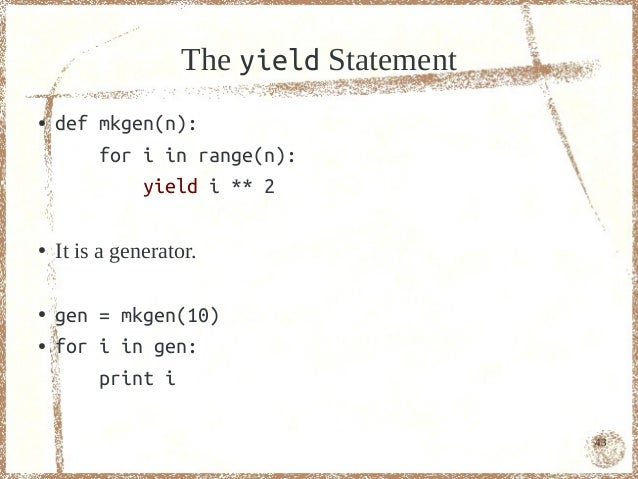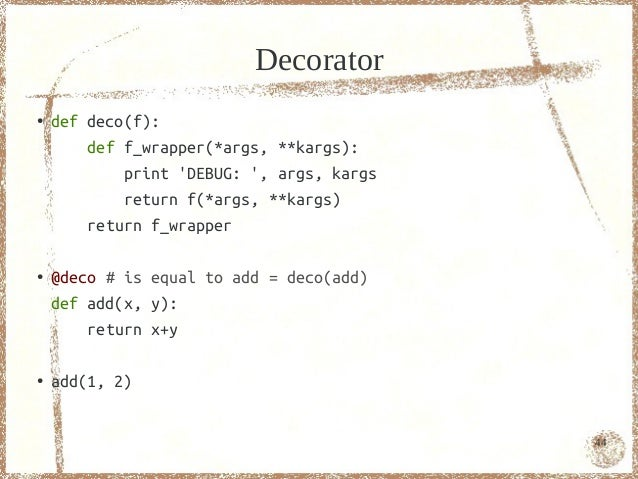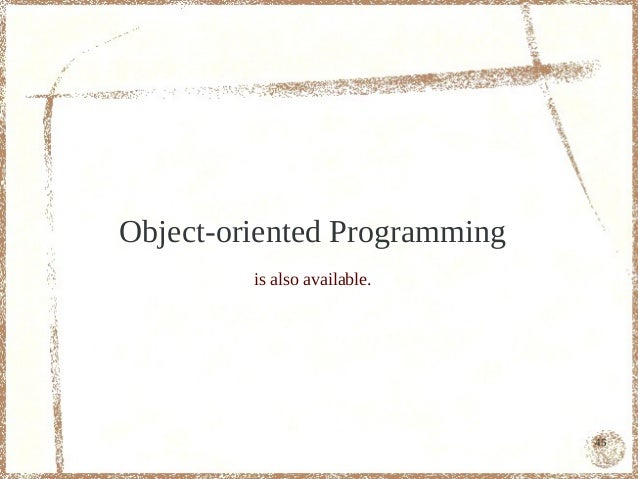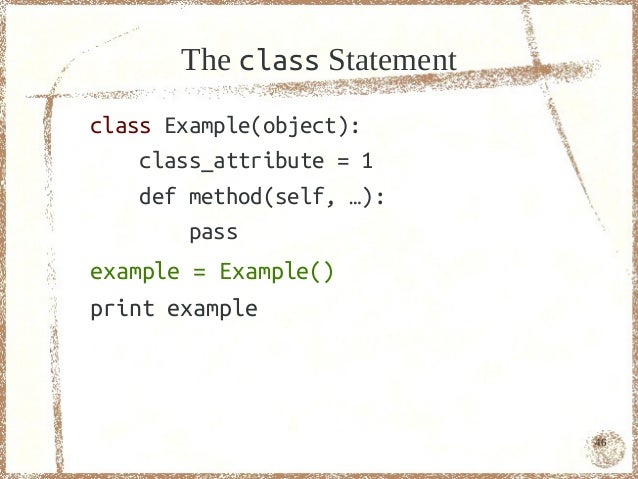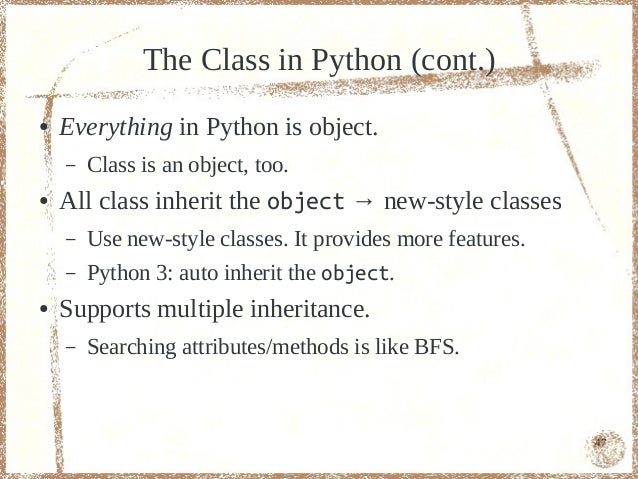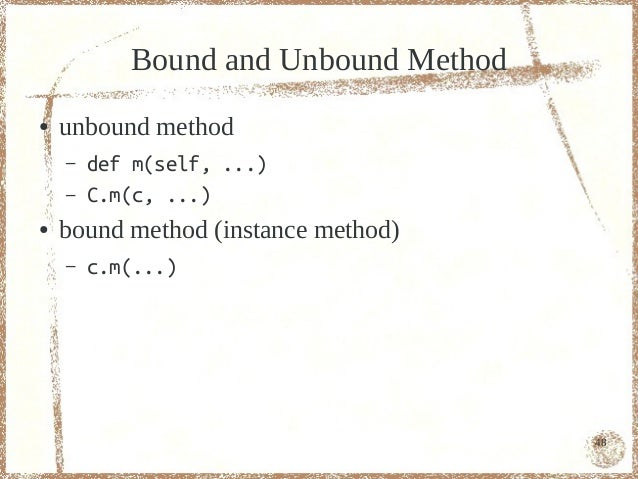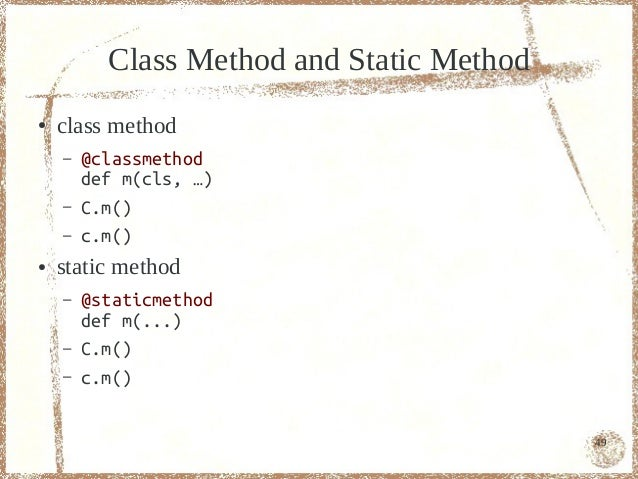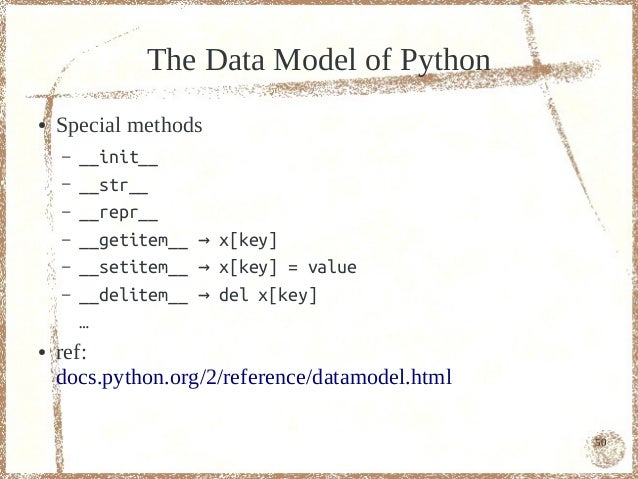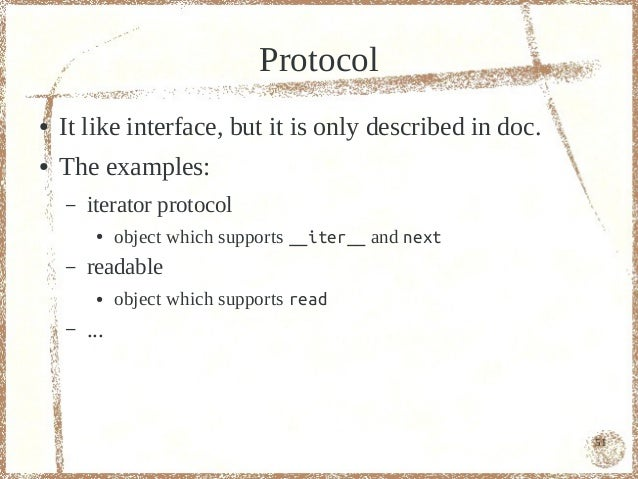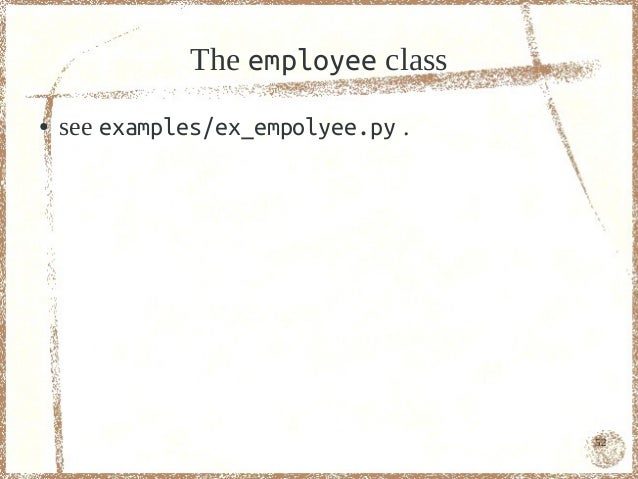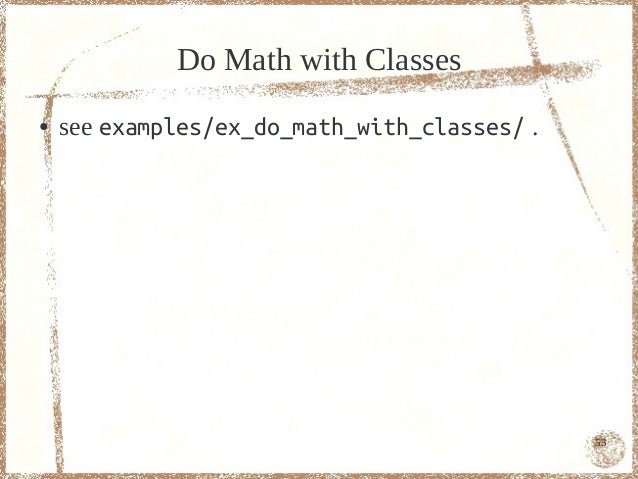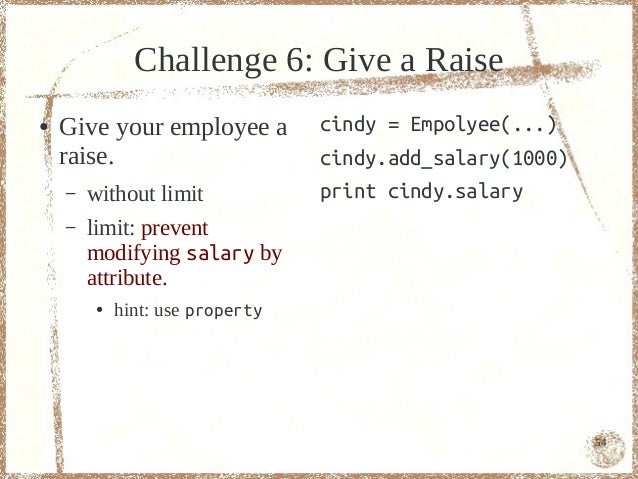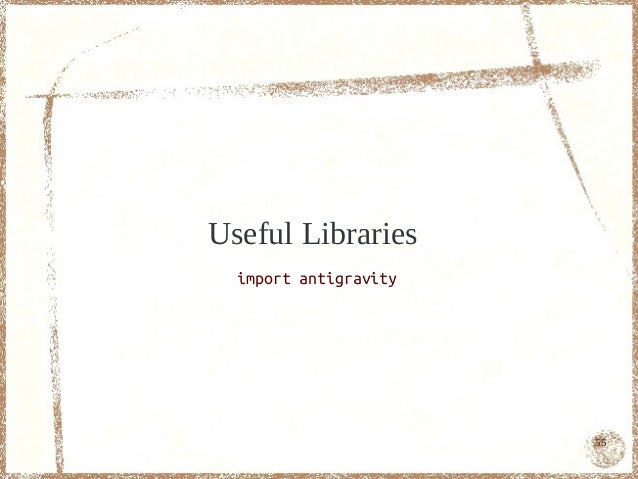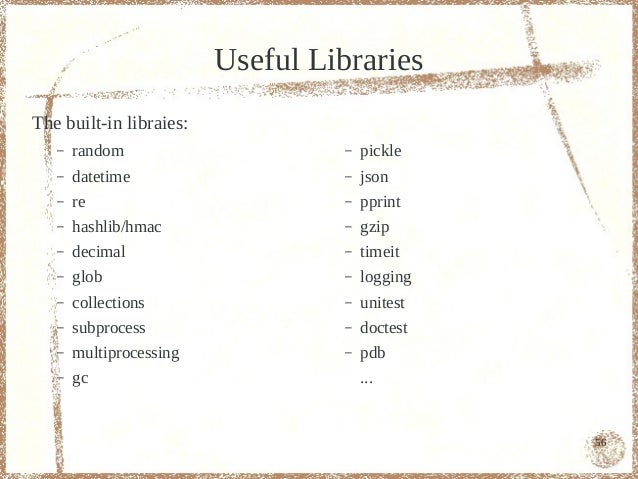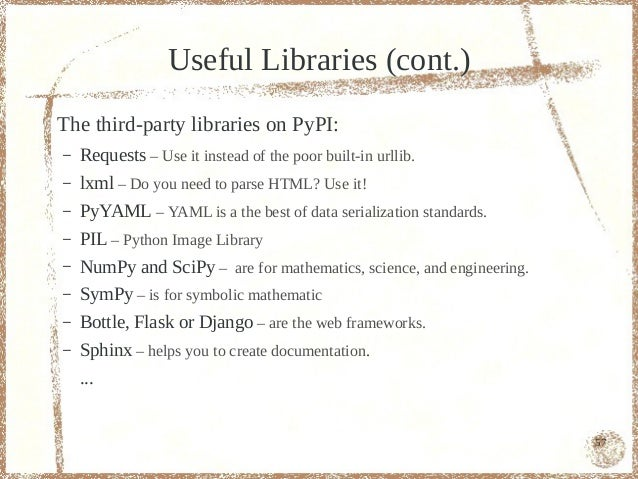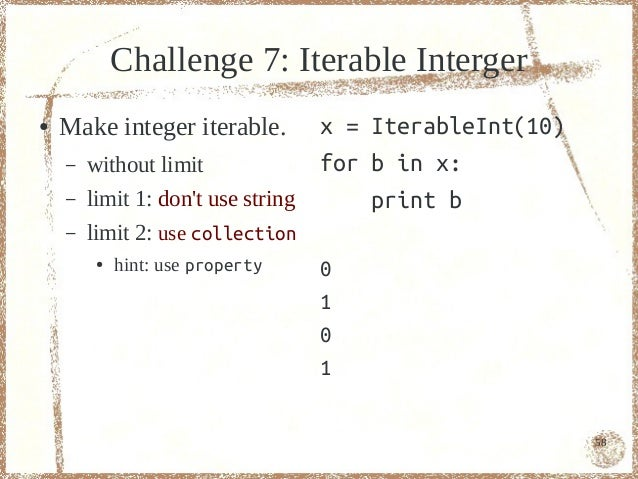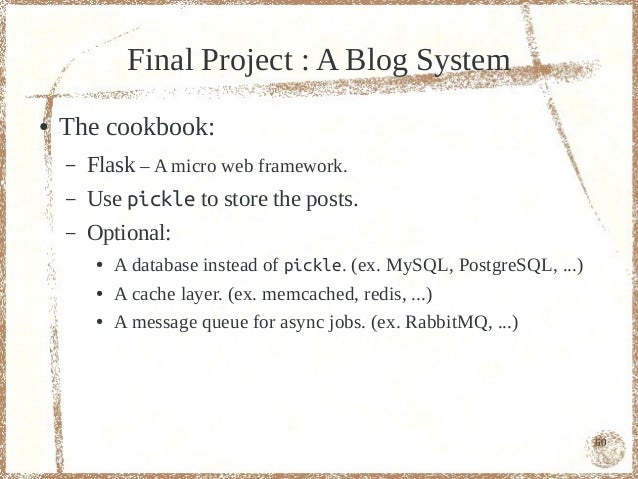Upcoming SlideShare
Loading in …5
×

# Programming with Python - Adv.

Python is a great programming language. It is a complete tutorial of using this programming language.

This slides is split into two parts, and it is the second part. Another part is at: http://www.slideshare.net/moskytw/programming-with-python-basic.

• Full Name
Comment goes here.

Are you sure you want to Yes No
Your message goes here• Be the first to comment

### Programming with Python - Adv.

1. 1. Programming with Python Adv. Topics Mosky 1
2. 2. Mosky:● The examples and the PDF version are available at: – j.mp/mosky-programming-with-python.● It is welcome to give me any advice of this slide or ask me the answers of the challenges. – mosky.tw 2
3. 3. Topics● Basic Topics ● Adv. Topics – Python 2 or 3? – Module and Package – Environment – Typing – hello.py – Comprehension – Common Types – Functional Technique – Flow Control – Object-oriented Prog. – File I/O – Useful Libraries – Documentation ● Final Project – Scope – A Blog System 3
4. 4. Module and PackageWrite you own module and package! 4
5. 5. Module and Package● A Python file is just a Python module: – import module_a # module_a.py● A folder which has __init__.py is just a Python package: – import package_x # __init__.py – import package_x.module_b # package_x/module_b.py – from . import module_c # (in package_x.moudle_b) package_x/module_c.py – \$ python -m package_x.module_b● Do not name your file as any built-in module. – ex. sys.py 5
6. 6. Module and Package (cont.)● The tree: – . ├── moudle_a.py └── package_x ├── __init__.py ├── module_b.py └── module_c.py 6
7. 7. The import Statement● A module is only imported at the first import.● import module module.val = modified – The module is affected by this modification.● from module import val val = modified – The module is not affected by this modification. – It does a shallow copy. 7
8. 8. Typingstatic? dynamic? weak? strong? 8
9. 9. Static Typing● Checking types in compile time.● Usually, it is required to give a type to a variable.● Python is not static typing. long x short y double z c1 c2 c3 c4 c5 c6 c7 c8 9
10. 10. Dynamic Typing● Checking types in run time.● A variable just points to an object.● Python is dynamic typing. x object A y object b object c NOTE: This is an animation and it is not correct in the PDF version. 10
11. 11. Duck Typing 11
12. 12. Duck Typing (cont.)● A style of dynamic typing.● Happy coding without the template, the generics … etc.● If it is necessary to check type: – if hasattr(x, __iter__): ● adapt the type inputed – assert not hasattr(x, __iter__), x must be iterable ● notify the programmer – if isinstance(x, basestring): ● the worst choice 12
13. 13. Duck Typing (cont.)#!/usr/bin/env python ● String and integer both# -*- coding: utf-8 -*-# file: ex_dyn.py support += operator.def dynsum(*seq): ● Write the code with r = seq for item in seq[1:]: elasticity. r += item return rif __name__ == __main__: print dynsum(1, 2, 3) print dynsum(x, y, z) 13
14. 14. Duck Typing (cont.)● BUT, it will confuse you when your project is going to big. – Name your variables with hint of type. ● item vs. items ● employee vs. employee_name ● args vs. kargs – Documentation does matter. 14
15. 15. Weak Typing● It converts the type if you do an operation which is not supported by the original type.● In JavaScript: – 1 + 1 → 11 – 1 == 1 → true● Python is not weak typing! 15
16. 16. Strong Typing● Only do the operations which are supported by the original type. – 1 + 1 → TypeError – 1 == 1 → False● Python is strong typing! 16
17. 17. ComprehensionCompact your statements. 17
18. 18. List Comprehension[i for i in range(10)][i ** 2 for i in range(10)][f(i) for i in range(10)][i for i in range(10) if i % 2 == 0][i for i in range(10) if not i % 2 == 0][i for i in range(10) if g(i)] 18
19. 19. List Comprehension (cont.)List comprehension: is equal to:[ r = [] (i, j) for i in range(3): for i in range(3) for j in range(3): for j in range(3) r.append((i, j))] 19
20. 20. List Comprehension (cont.)List comprehension: is equal to:[ r = [] [ for i in range(3): (i, j) t = [] for i in range(3) for j in range(3): ] t.append((i, j)) for j in range(3) r.append(t)] 20
21. 21. Generator Comprehension● Generator comprehension: – The examples: ● (i for i in range(10)) ● f(i for i in range(10)) – It is like xrange. – Lazy evaluation → Save memory. 21
22. 22. Other ComprehensionsPython 3 only:● set comprehension: – {i for i in range(10)}● dict comprehension: – {i:i for i in range(10)}But we can do so with below statements:● set comprehension: – set(i for i in range(10))● dict comprehension: – dict((i, i) for i in range(10)) 22
23. 23. Functional Technique Think in the functional way. 23
24. 24. The any/all Function● def all_even(seq): return all(i % 2 == 0 for i in seq)● all(type(item) is int for item in inputs)● any(i < 0 for i in inputs) 24
25. 25. Challenge 3-3: The Primes (cont.)– limit: in one line. [2, 3, 5, 7, 11, 13, ● hint: use any or all 17, 19, 23, 29, 31, 37, 41, 43, 47, 53, 59, 61, 67, 71, 73, 79, 83, 89, 97] 25
26. 26. The zip Function● matrix = [ [1, 2], [3, 4], ]● zip(*matrix) 26
27. 27. The zip Function (cont.)● result = [ (I am A., email_A), (I am B., email_B), ]● emails = zip(*result) 27
28. 28. First-class Functions#!/usr/bin/env python ● passing functions as# -*- coding: utf-8 -*-# file: ex_do.py arguments.from operator import add, muldef do(action, x, y): return action(x, y)if __name__ == __main__: print do(add, 10, 20) print do(mul, 10, 20) 28
29. 29. The lambda Expression● lambda [args]: [expression]● It defines an anonymous function.● It only allows a single expression.● f = lambda x: g(x)+h(x)● do(lambda x, y: (x+y)*(x+y), 10, 20) 29
30. 30. Use sort with Lambda● d = dict(a=300, b=200, c=100)● keys = d.keys()● keys.sort(key=lambda k: d[k])● for k in keys: print k, d[k] 30
31. 31. Use sort with Lambda (cont.)● names = [Andy, Bob, Cindy]● scores = [70, 100, 95]● table = zip(names, scores)● table.sort(key=lambda pair: pair)● for name, score in table: print name, score 31
32. 32. The map Function● map(lambda x: x**2, range(10))● map(int, 1 2 3.split( ))● map(ord, String)● map(open, [<paths>])● map(str.split, open(<path>)) 32
33. 33. The map Function (cont.)● from operator import mul● a = (1, 2)● b = (3, 4)● sum(map(mul, a, b)) 33
34. 34. The filter Function● filter(lambda i: i % 2 == 0, range(10))● filter(str.isdigit, strings)● filter(lambda s: s.endswith(.py), file_names) 34
35. 35. Comprehension vs. map/filter● [i ** 2 for i in range(10)]● map(lambda i: i ** 2, range(10))● [i ** 2 for i in range(10) if i % 2 == 0]● map(lambda i: i ** 2, filter( lambda i: i % 2 == 0, range(10) )) 35
36. 36. Comprehension vs. map/filter (cont.)● [ord(c) for c in ABC]● map(ord, ABC) 36
37. 37. Comprehension vs. map/filter (cont.) Compare the speeds of them: 1. map/filter (with built-in function) 2. comprehension 3. map/filter 37
38. 38. The reduce Function● # from functools import reduce # py3● from operator import add● seq = [-1, 0, 1]● reduce(add, s)● seq = [reduce , the , lines.]● reduce(add, s) 38
39. 39. The partial Function● from functools import partial● from operator import add● rdsum = partial(reduce, add)● rdsum([-1, 0, 1])● rdsum([reduce , the , lines.]) 39
40. 40. The partial Function (cont.)● from functools import partial● from fractions import gcd as _gcd● _gcd(6, 14)● gcd = partial(reduce, _gcd)● gcd([6, 14, 26]) 40
41. 41. Closure● from math import log● def mklog(n): return lambda x: log(x, n)● log10 = mklog(10)● log10(100) # n = 10 41
42. 42. Closure (cont.)● setattr(DictLike, attrname, # it is a colsure (lambda x: property( lambda self: self.__getitem__(x), lambda self, v: self.__setitem__(x, v), lambda self: self.__delitem__(x) ) )(attrname) ) 42
43. 43. The yield Statement● def mkgen(n): for i in range(n): yield i ** 2● It is a generator.● gen = mkgen(10)● for i in gen: print i 43
44. 44. Decorator● def deco(f): def f_wrapper(*args, **kargs): print DEBUG: , args, kargs return f(*args, **kargs) return f_wrapper● @deco # is equal to add = deco(add) def add(x, y): return x+y● add(1, 2) 44
45. 45. Object-oriented Programming is also available. 45
46. 46. The class Statementclass Example(object): class_attribute = 1 def method(self, …): passexample = Example()print example 46
47. 47. The Class in Python (cont.)● Everything in Python is object. – Class is an object, too.● All class inherit the object → new-style classes – Use new-style classes. It provides more features. – Python 3: auto inherit the object.● Supports multiple inheritance. – Searching attributes/methods is like BFS. 47
48. 48. Bound and Unbound Method● unbound method – def m(self, ...) – C.m(c, ...)● bound method (instance method) – c.m(...) 48
49. 49. Class Method and Static Method● class method – @classmethod def m(cls, …) – C.m() – c.m()● static method – @staticmethod def m(...) – C.m() – c.m() 49
50. 50. The Data Model of Python● Special methods – __init__ – __str__ – __repr__ – __getitem__ → x[key] – __setitem__ → x[key] = value – __delitem__ → del x[key] …● ref: docs.python.org/2/reference/datamodel.html 50
51. 51. Protocol● It like interface, but it is only described in doc.● The examples: – iterator protocol ● object which supports __iter__ and next – readable ● object which supports read – ... 51
52. 52. The employee class● see examples/ex_empolyee.py . 52
53. 53. Do Math with Classes● see examples/ex_do_math_with_classes/ . 53
54. 54. Challenge 6: Give a Raise● Give your employee a cindy = Empolyee(...) raise. cindy.add_salary(1000) – without limit print cindy.salary – limit: prevent modifying salary by attribute. ● hint: use property 54
55. 55. Useful Libraries import antigravity 55
56. 56. Useful LibrariesThe built-in libraies: – random – pickle – datetime – json – re – pprint – hashlib/hmac – gzip – decimal – timeit – glob – logging – collections – unitest – subprocess – doctest – multiprocessing – pdb – gc ... 56
57. 57. Useful Libraries (cont.)The third-party libraries on PyPI:– Requests – Use it instead of the poor built-in urllib.– lxml – Do you need to parse HTML? Use it!– PyYAML – YAML is a the best of data serialization standards.– PIL – Python Image Library– NumPy and SciPy – are for mathematics, science, and engineering.– SymPy – is for symbolic mathematic– Bottle, Flask or Django – are the web frameworks.– Sphinx – helps you to create documentation. ... 57
58. 58. Challenge 7: Iterable Interger● Make integer iterable. x = IterableInt(10) – without limit for b in x: – limit 1: dont use string print b – limit 2: use collection ● hint: use property 0 1 0 1 58
59. 59. Final Project : A Blog System 59
60. 60. Final Project : A Blog System● The cookbook: – Flask – A micro web framework. – Use pickle to store the posts. – Optional: ● A database instead of pickle. (ex. MySQL, PostgreSQL, ...) ● A cache layer. (ex. memcached, redis, ...) ● A message queue for async jobs. (ex. RabbitMQ, ...) 60
61. 61. It is the end.Contact me? http://mosky.tw/ 61